Home > CCA2 > Chapter 9 > Lesson 9.2.1 > Problem9-58

9-58.
1. Solve each equation. Homework Help ✎

1.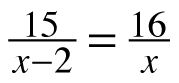2.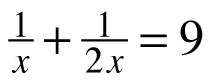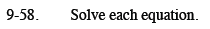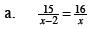Multiply both sides of the equation by (x) and (x − 2).

15x = 16(x − 2)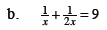$x=\frac{1}{6}$